# The GAMPL Procedure

### Model Inference

Wahba (1983) proposes a Bayesian covariance matrix for parameter estimates
by interpreting a smoothing spline as a posterior mean. Nychka (1988) shows that the derived Bayesian posterior confidence limits work well from frequentist viewpoints. The Bayesian posterior covariance matrix for the parameters is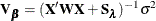The posterior distribution foris thus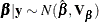For a particular point whose design row is vector, the prediction is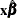and the standard error is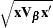. The Bayesian posterior confidence limits are thus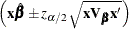where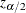is the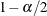quantile of the standard normal distribution.

For the jth spline term, the prediction for the component is formed by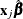, where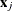is a row vector of zeros except for columns that correspond to basis expansions of the jth spline term. And the standard error for the component is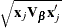.